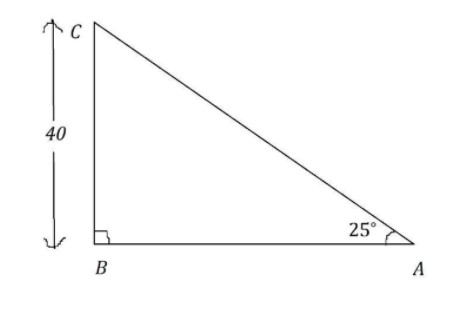QuestionAnswers

# A boat sights the top of a $40$ foot lighthouse at an angle of elevation of $25$ degrees. Calculate the distance of the boat from the lighthouse, the horizontal distance to the nearest tenth of a foot.

Hint:This problem is based on the applications of trigonometry. Draw the diagram according to the data given. Then after you are able to see the right-angled triangle in the diagram. Apply basic trigonometric formulae to get the solution.

A boat sights the top of the lighthouse.
The height of the lighthouse is $40$ foot.
The angle of elevation is ${25^ \circ }$.
Let us draw the diagram as per the above data. It will look as follows:From the diagram,
At $A$ the boat is present and the $BC$ is the height of the lighthouse.
Consider the $\vartriangle ABC$,
In order to calculate the horizontal distance between the boat and lighthouse we need to calculate the length of $AB$.
Let us apply the $\tan \theta$ in $\vartriangle ABC$ at $A$.
We know the formula of $\tan \theta = \dfrac{{{\text{Opposite}}}}{{Adjacent}}$
So, in $\vartriangle ABC$
$\Rightarrow \tan A = \dfrac{{BC}}{{AB}}$
Substitute the values $A = {25^ \circ }$and $BC = 40$
$\Rightarrow \tan {25^ \circ } = \dfrac{{40}}{{AB}}$
Find the value of $AB$ from the above equation.
$\Rightarrow AB = \dfrac{{40}}{{\tan {{25}^ \circ }}}$
Substitute the value of $\tan {25^ \circ } = 0.46$
If you don’t know the exact value of $\tan {25^ \circ } = 0.46$, you can still get the answer by predicting the approximate value of it from analyzing its graph.
$\ \Rightarrow AB = \dfrac{{40}}{{0.46}} \\ \Rightarrow AB = 86.95 \\ \$
So, the horizontal distance from the boat to the lighthouse is $87$ foot approximately.

Note:When you read this kind of problem, your next step after clear understanding is to draw the diagram. When you are mentioning the parameters be sure that you are not doing anything in the wrong way. Be aware while you are calculating, because sometimes the units of the measurements will be different.